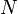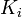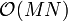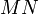# Simulation

(diff) ← Older revision | Latest revision (diff) | Newer revision → (diff)

Simulation is a technique in programming where all events are processed in the order of occurrence. By translating real world logic into programming code, simulation speeds up tasks that may otherwise take a long time for human. Simulation does not provide alternative shortcuts to a solution; it simply speeds up the process.

## Example

### Problem

Given$M$ trees and the number of days each tree takes to grow a fruit, compute the total number of fruits after$N$ days.

### Solution

For each tree$i$, keep a variable$K_i$ indicating the number of days it takes for the next fruit to grow. Simulate the situation day by day. On each day, decrease$K_i$ by 1 and increase the fruit count by the number of$K_i$'s that equal zero, and reset these$K_i$'s. Output the total sum at the end.

### Analysis

This solution gives the correct answer, in$\mathcal{O}(MN)$ time. This is fine if$MN$ is small, but a better technique is required if$MN$ is large.

## Characteristics

When a problem is logically simple and can be directly done by human, simulation is very likely the solution. When the bounds on variables are small, simulation is a quick, safe, and convincing technique. When run time is a concern, other techniques such as dynamic programming may be required.